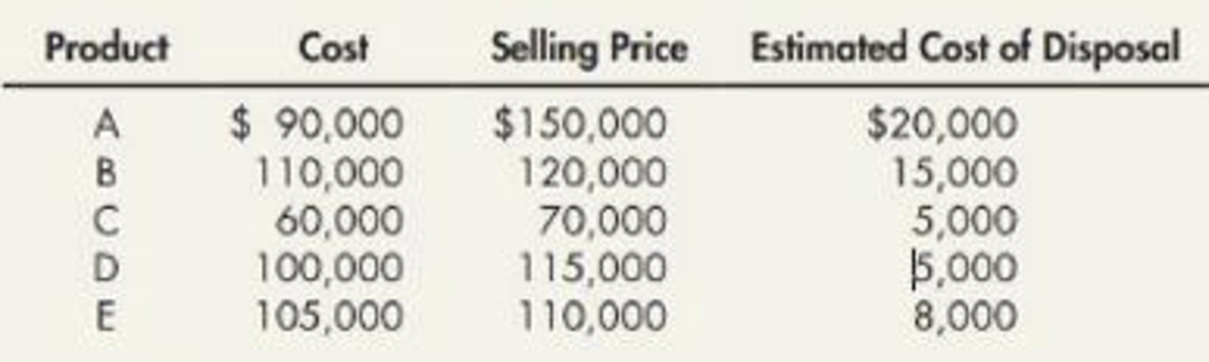Chapter 8, Problem 3E### Intermediate Accounting: Reporting...

3rd Edition
James M. Wahlen + 2 others
ISBN: 9781337788281

#### Solutions

Chapter
Section### Intermediate Accounting: Reporting...

3rd Edition
James M. Wahlen + 2 others
ISBN: 9781337788281
Textbook Problem
535 views

# Inventory Write-Down Byron Company has five products in its inventory and uses the FIFO cost flow assumption. Specific data for each product are as follows:Required: 1. What is the correct inventory value, assuming the LCNRV rule is applied to each item of inventory? 2. What is the correct inventory value, assuming the LCNRV rule is applied to the total of inventory? 3. Next Level Comment on any differences that result from applying the LCNRV rule to individual items compared to the total of inventory.

1.

To determine

Calculate the correct value of inventory if company uses LCNRV rule to each item of inventory.

Explanation

Lower-of-cost-or-market: The lower-of-cost-or-market (LCM) is a method which requires the reporting of the ending merchandise inventory in the financial statement of a company, either at current market value or at historical cost price of the inventory, whichever is less.

NRV (Net Realizable Value): It refers to an estimated selling price that a company expects to collect in the form of cash from the customers by the sale of inventory...

2.

To determine

Calculate the correct value of inventory if company uses LCNRV rule to the total of inventory.

3.

To determine

Indicate any differences that result from the use of LCNRV rule to each item of inventory when compared to the total of inventory.

### Still sussing out bartleby?

Check out a sample textbook solution.

See a sample solution

#### The Solution to Your Study Problems

Bartleby provides explanations to thousands of textbook problems written by our experts, many with advanced degrees!

Get Started

#### Find more solutions based on key concepts